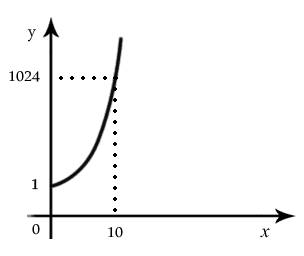# 【随笔】什么是函数？x  –> [ f ] –> f(x) –> [ g ] — g(f(x))

g(f(x)) 即 f 和 g 的复合函数。

y = f(x)

f(4) = 40亿元 ,

(f( 4 + e) – f(4)) / e = g(4)

(f( 4 + e) – f(4)) / e 可以通过下面的变化转为近似函数g(x)：

=(20√4+e – 20√4) / e

=(20√4+e – 20 * 2) / e

=20* ( ((( (√4+e)-2) * ( (√4+e)+2) ) / e * (( (√4+e)+2 ) )

=20 / ((√4+e) + 2)

g(x) 中x的系数为5， 表示播放时长每增加1小时，就会带来5亿元的收入，所以仅增加6分钟的话，就会带来0.5亿来的收入。同时，广告时间6分钟需要6 * 0.1 = 0.6亿元广告费。